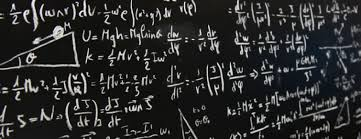Algebra Level 5How many ordered triples of real numbers $(a, b, c)$ are there which satisfy:

$a^{2} + b^{2} +c^{2} = 66$ $a^{3} + b^{3} + c^{3} = 408$$a^{4} + b^{4} + c^{4} = 2658$ This is part of Ordered Disorder.

×